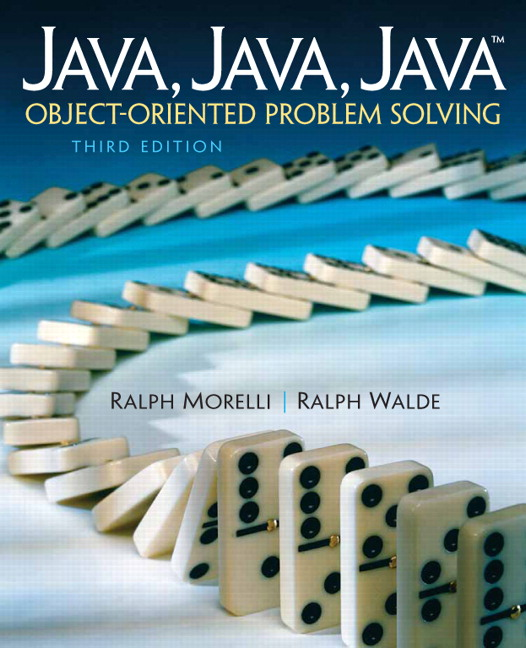# Java, Java, Java: Object-Oriented Problem Solving, 2022E

## Section6.12Special Topic: What Can Be Computed?

Did you ever wonder whether there are problems that cannot be solved by a computer, no matter what kind of control structures are used? Well, back in 1939, in his seminal paper titled “On Computable Numbers,” Alan Turing proved that indeed there are an infinite number of unsolvable problems. Prior to this, mathematicians and logicians thought all problems could be solved. So Turing’s proof was quite a blow!
To help him prove this point, Turing defined an abstract computer, which has come to be known as a Turing machine. A Turing machine has an alphabet of symbols; a read/write head; an infinitely long tape on which the read/write head can write symbols, and from which it can also read symbols; and a control unit, which controls the movement and action of the read/write head. Note that the Turing machine elements correspond to key components of a real computer—although Turing invented this concept a decade before the first computers were developed. The read/write head corresponds to a computer’s central processing unit (CPU). The tape corresponds to the computer’s memory. And the control unit corresponds to the computer program.
A Turing machine represents a purely abstract concept of computation. It represents the pure idea of an algorithmic solution to a problem. Equipped with this concept, Turing was able to prove that there are unsolvable problems—that is, problems for which no algorithm can arrive at a solution.
One such problem is the halting problem. This problem asks whether an algorithm can be devised to determine whether an arbitrary program will eventually halt. If there were such an algorithm, it could be used to detect programs that contain infinite loops, a service that might be really helpful in an introductory computing lab, among other places! But, alas, there can be no such algorithm.
Here’s an outline of a proof that shows that the halting problem is unsolvable. (This particular version of the proof was suggested by J. Glenn Brookshear in Computer Science: An Overview, Benjamin-Cummings, 1985.)
Suppose you had a program, P, that solves the halting problem. That is, whenever P is given a self-halting program, it sets a variable isTerminating to true, and otherwise it sets isTerminating to false. Now let’s create a new version of P, named $$P\prime\text{,}$$ which is identical to P except that right after where P sets isTerminating to true or false, $$P\prime$$ contains the following loop:
while (isTerminating == true);  // Infinite if isTerminating true

In other words, if the input to $$P\prime$$ is a self-terminating program, then $$P\prime$$ will enter an infinite loop and it won’t terminate. Otherwise, if a non-self-terminating program is input to $$P\prime\text{,}$$ $$P\prime$$ will skip the loop and will terminate.
Now what if we give a representation of $$P\prime$$ to itself. Will it halt? The answer generates a contradiction: If $$P\prime$$ is a self-terminating program, then when it is input to itself, it will not terminate. And if $$P\prime$$ is not self-terminating, when it is input to itself, it will terminate. Because our assumption that P solves the halting problem has led to a contradiction, we have to conclude that it wasn’t a very good assumption in the first place. Therefore, there is no program that can solve the halting problem.
The topic of computability is a fundamental part of the computer science curriculum, usually taught in a sophomore- or junior-level theory of computation course.